Editor's Note: This article was translated and edited by SAS USA and was originally written by Makoto Unemi. The original text is here.

SAS previously provided SAS Scripting Wrapper for Analytics Transfer (SWAT), a package for using SAS Viya functions from various general-purpose programming languages ​​such as Python.

In addition to SWAT, SAS launched Deep Learning Python (DLPy), a higher-level API package for Python, making it possible to use SAS Viya functions more efficiently from Python. In this article I outline more about what DLPy is and how it's implementation.

DLPy is a high-level package for the Python API created for deep learning and image action set after Viya3.3. DLPy provides an API similar to Keras to improve the efficiency of deep learning and image processing coding. With just a little rewriting of the existing Keras code, it is possible to execute the processing on SAS Viya.

For example, below is an example of a Convolutional Neural Network (CNN) layer definition; you can see that it is very similar to Keras.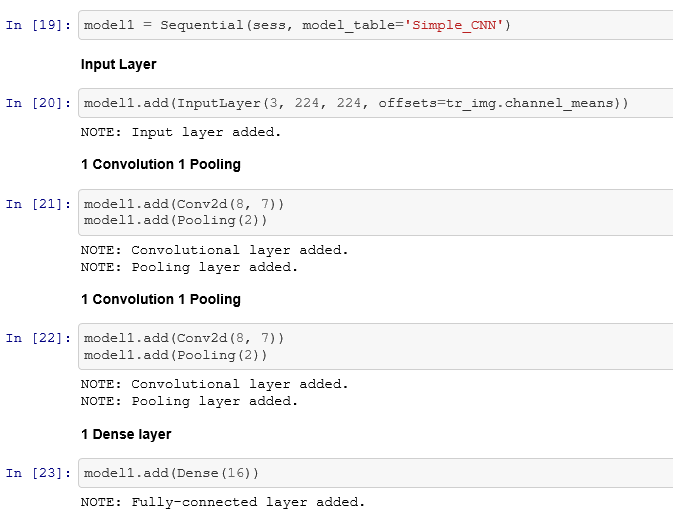The layers supported by DLPy are: InputLayer, Conv2d, Pooling, Dense, Recurrent, BN, Res, Proj, and OutputLayer. The following is an example of learning.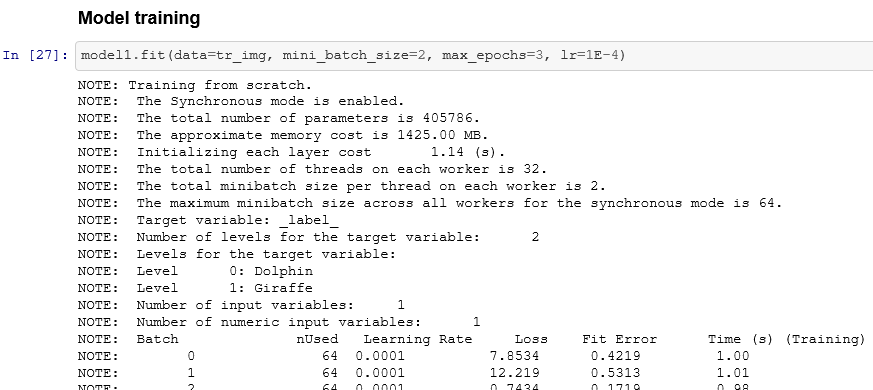## DLPy functions

Introducing DLPy's functions (partial excerpts), taking as an example the learning of multiple dolphins and giraffe images using CNN and applying test images to the model.

### Implementation of major deep learning networks

DLPy offers the following pre-built deep learning models: VGG11/13/16/19, ResNet34/50/101/152, wide_resnet, and dense_net.

The following models also offer pre-trained weights using ImageNet data (these weights can be used for unique tasks by transfer learning): VGG16, VGG19, ResNet50, ResNet101, and ResNet152. The following is an example of transferring ResNet50 pre-trained weights.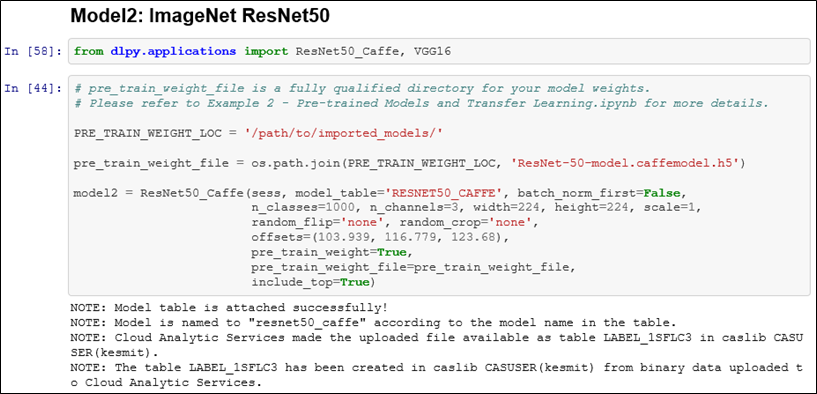### CNN judgment basis information

Using the `heat_map_analysis()` method, you can output a colorful heat map and check where you focused on the image.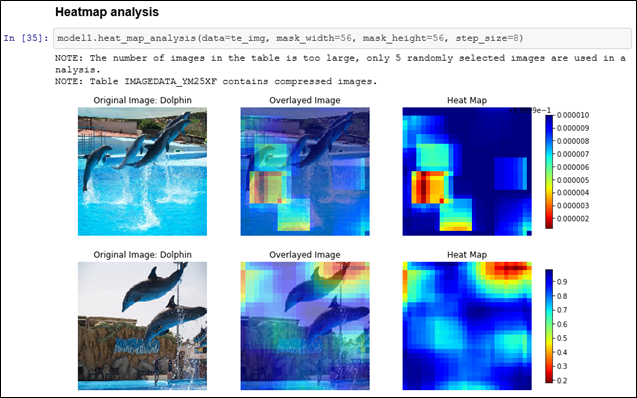In addition, the `get_feature_maps()` method is used to get the feature map of each layer of CNN, and `feature_maps.display()` method is used to specify and display the obtained feature map layer and check can also do.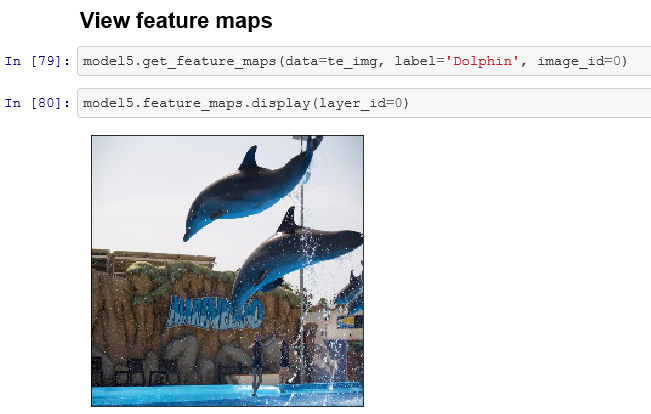The following is the output result of layer 1 feature map.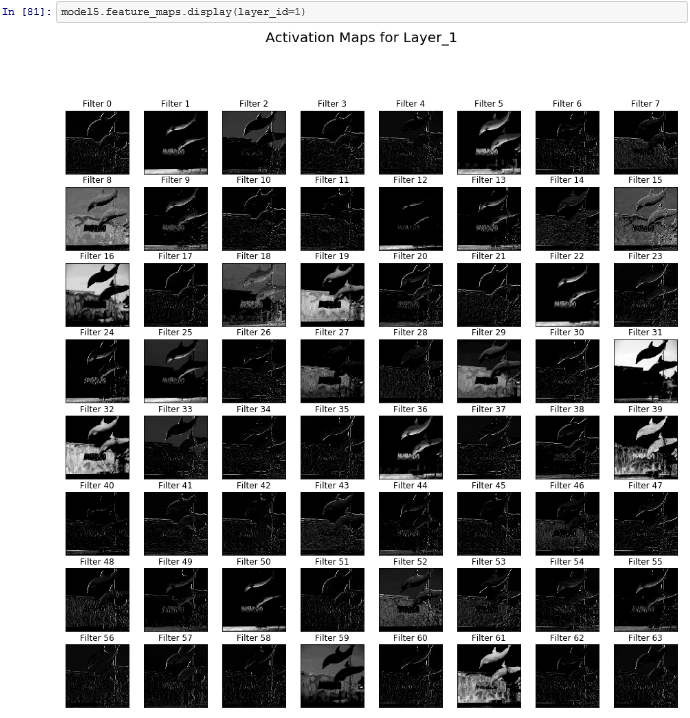The following is the output result of layer 18 feature map.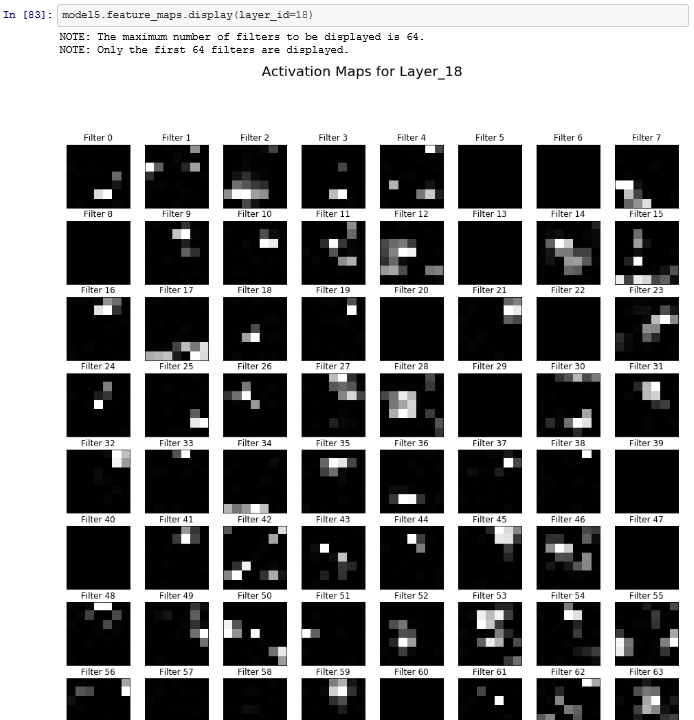### Deep learning & image processing related task support function

#### `resize()` method: Resize image data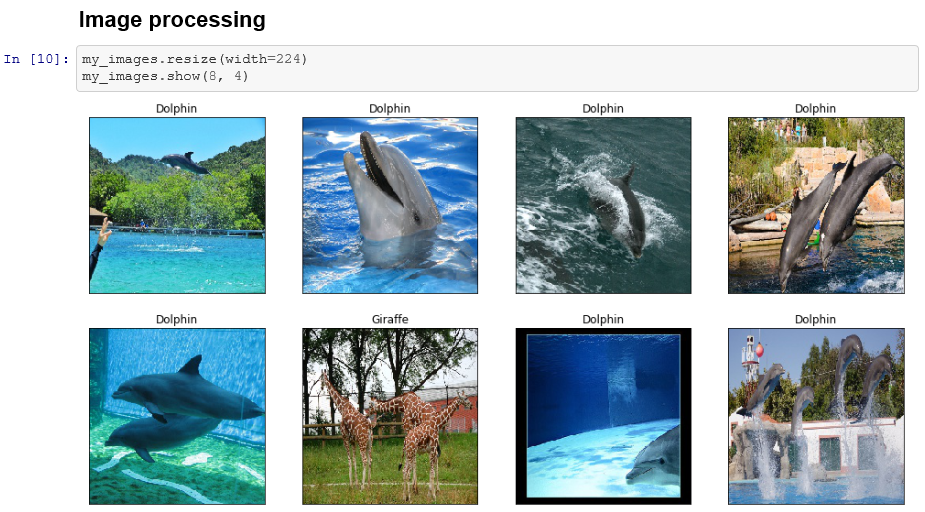#### `as_patches()` method: Image data expansion (generates a patch from the original image)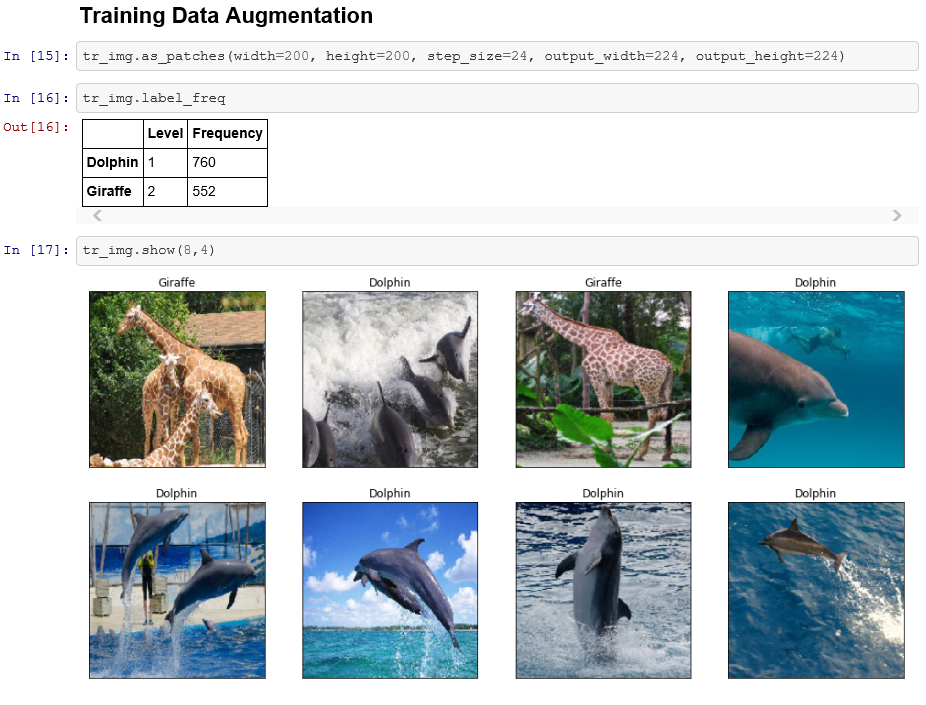#### `two_way_split()` method: Data split (learning, testing)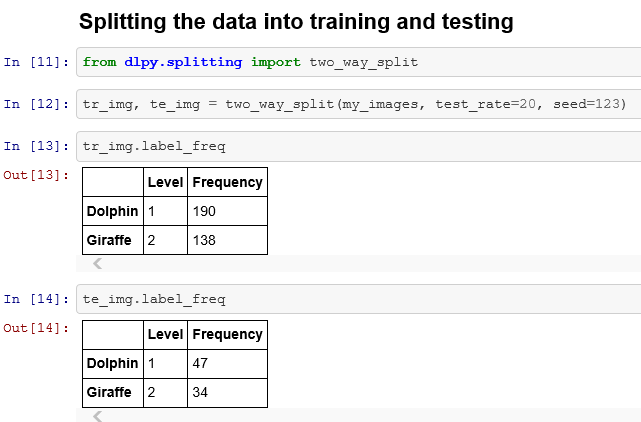#### `plot_network()` method: draws the structure of the defined deep learning layer (network) as a graphical diagram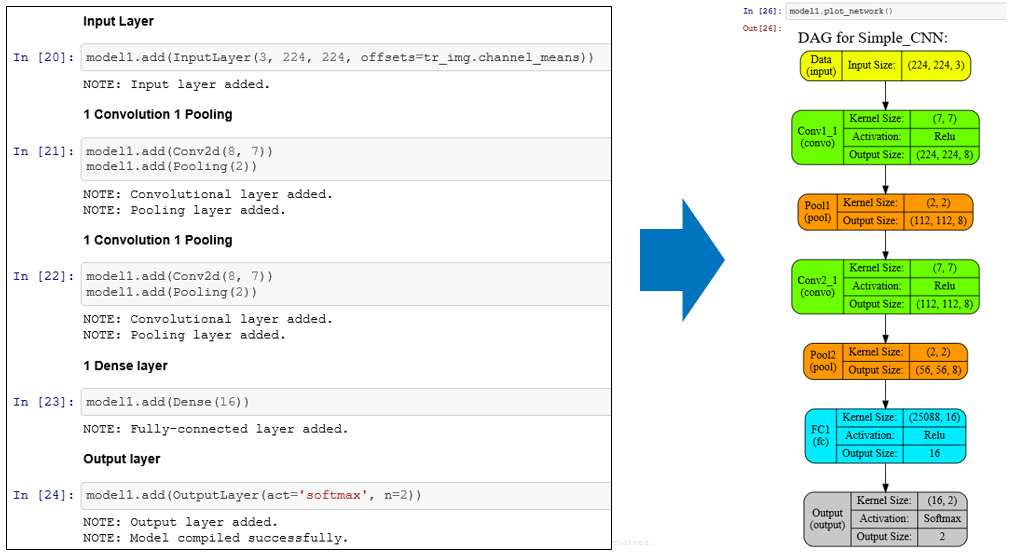#### `plot_training_history()` method: Iterative learning history display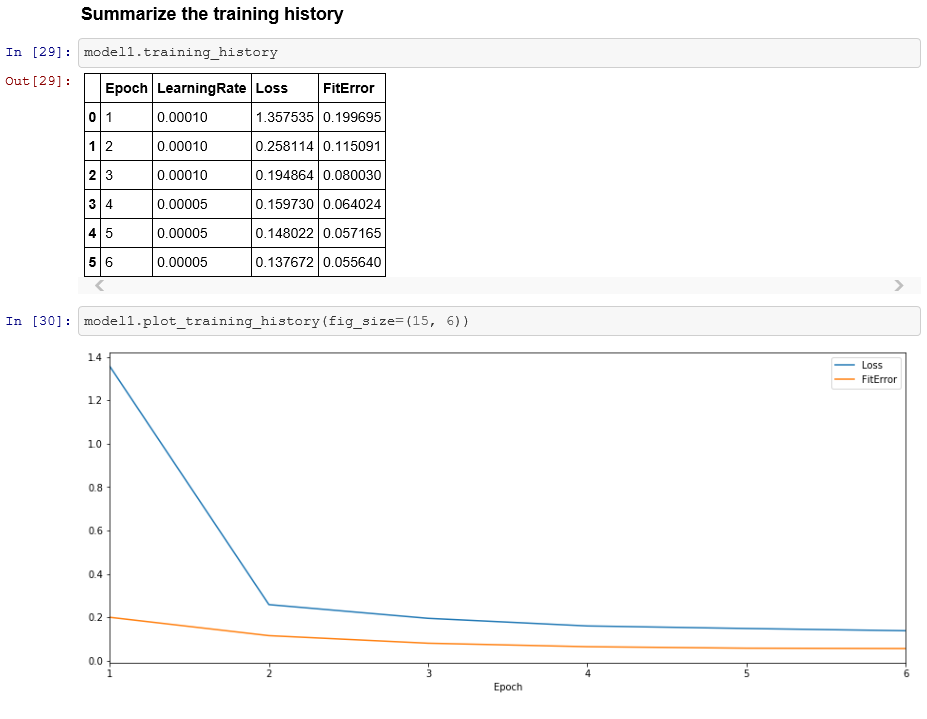#### `predict()` method: Display prediction (scoring) results#### `plot_predict_res()` method: Display classification results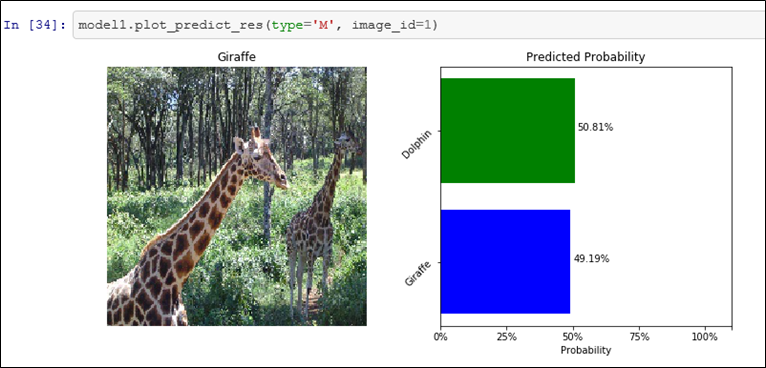And of course, you can use DLPy to get data from a SAS Viya in-memory session, pass it to your local client, and convert it to common data formats like numpy arrays and Pandas DataFrames. The converted data can be smoothly supplied to models of other open source packages such as scikit-learn.

Regarding image classification using DLPy, videos are also available in the Deep Learning with Python (DLPy) Demo Series section of the DLPy product page.According to the World Cancer Research Fund, Breast cancer is one of the most common cancers worldwide, with 12.3% of new cancer patients in 2018 suffering from breast cancer. Early detection can significantly improve treatment value, however, the interpretation of cancer images heavily depends on the experience of doctors and technicians. The [...]Artificial intelligence (AI) is a natural evolution of analytics. Over the years, we have seen AI add learning and automation capabilities to the predictive and prescriptive jobs of analytics. We have been building AI systems for decades, but a few things have changed to make today’s AI systems more powerful. [...]

Advancing AI with deep learning and GPUs was published on SAS Voices by Oliver SchabenbergerArtificial intelligence (AI) is a natural evolution of analytics. Over the years, we have seen AI add learning and automation capabilities to the predictive and prescriptive jobs of analytics. We have been building AI systems for decades, but a few things have changed to make today’s AI systems more powerful. [...]

Advancing AI with deep learning and GPUs was published on SAS Voices by Oliver SchabenbergerDeep learning (DL) is a subset of neural networks, which have been around since the 1960’s. Computing resources and the need for a lot of data during training were the crippling factor for neural networks. But with the growing availability of computing resources such as multi-core machines, graphics processing units (GPUs) accelerators and hardware specialized, DL is becoming much more practical for business problems.

Financial institutions use a large number of computations to evaluate portfolios, price securities, and financial derivatives. For example, every cell in a spreadsheet potentially implements a different formula. Time is also usually of the essence so having the fastest possible technology to perform financial calculations with acceptable accuracy is paramount.

In this blog, we talk to Henry Bequet, Director of High-Performance Computing and Machine Learning in the Finance Risk division of SAS, about how he uses DL as a technology to maximize performance.

Henry discusses how the performance of numerical applications can be greatly improved by using DL. Once a DL network is trained to compute analytics, using that DL network becomes drastically faster than more classic methodologies like Monte Carlo simulations.

We asked him to explain deep learning for numerical analysis (DL4NA) and the most common questions he gets asked.

### Can you describe the deep learning methodology proposed in DL4NA?

Yes, it starts with writing your analytics in a transparent and scalable way. All content that is released as a solution by the SAS financial risk division uses the "many task computing" (MTC) paradigm. Simply put, when writing your analytics using the many task computing paradigm, you organize code in SAS programs that define task inputs and outputs. A job flow is a set of tasks that will run in parallel, and the job flow will also handle synchronization.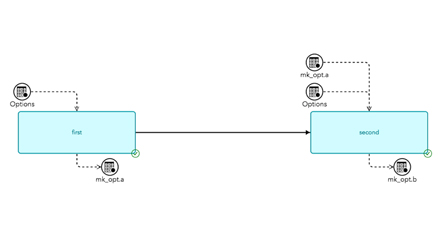Fig 1.1 A Sequential Job Flow

The job flow in Figure 1.1 visually gives you a hint that the two tasks can be executed in parallel. The addition of the task into the job flow is what defines the potential parallelism, not the task itself. The task designer or implementer doesn’t need to know that the task is being executed at the same time as other tasks. It is not uncommon to have hundreds of tasks in a job flow.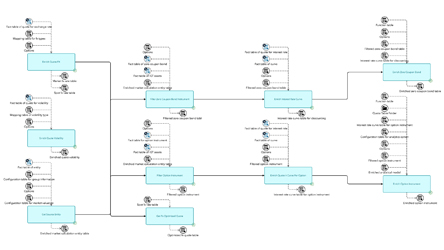Fig 1.2 A Complex Job Flow

Using that information, the SAS platform, and the Infrastructure for Risk Management (IRM) is able to automatically infer the parallelization in your analytics. This allows your analytics to run on tens or hundreds of cores. (Most SAS customers run out of cores before they run out of tasks to run in parallel.) By running SAS code in parallel, on a single machine or on a grid, you gain orders of magnitude of performance improvements.

This methodology also has the benefit of expressing your analytics in the form of Y= f(x), which is precisely what you feed a deep neural network (DNN) to learn. That organization of your analytics allows you to train a DNN to reproduce the results of your analytics originally written in SAS. Once you have the trained DNN, you can use it to score tremendously faster than the original SAS code. You can also use your DNN to push your analytics to the edge. I believe that this is a powerful methodology that offers a wide spectrum of applicability. It is also a good example of deep learning helping data scientists build better and faster models.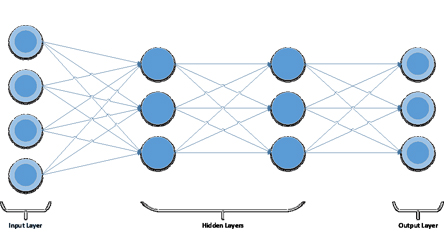Fig 1.3 Example of a DNN with four layers: two visible layers and two hidden layers.

The number of neurons of the input layer is driven by the number of features. The number of neurons of the output layer is driven by the number of classes that we want to recognize, in this case, three. The number of neurons in the hidden layers as well as the number of hidden layers is up to us: those two parameters are model hyper-parameters.

## How do I run my SAS program faster using deep learning?

In the financial risk division, I work with banks and insurance companies all over the world that are faced with increasing regulatory requirements like CCAR and IFRS17. Those problems are particularly challenging because they involve big data and big compute.

The good news is that new hardware architectures are emerging with the rise of hybrid computing. Computers are increasing built as a combination of traditional CPUs and innovative devices like GPUs, TPUs, FPGAs, ASICs. Those hybrid machines can run significantly faster than legacy computers.

The bad news is that hybrid computers are hard to program and each of them is specific: you write code for GPU, it won’t run on an FPGA, it won’t even run on different generations of the same device. Consequently, software developers and software vendors are reluctant to jump into the fray and data scientist and statisticians are left out of the performance gains. So there is a gap, a big gap in fact.

To fill that gap is the raison d’être of my new book, Deep Learning for Numerical Applications with SAS. Check it out and visit the SAS Risk Management Community to share your thoughts and concerns on this cross-industry topic.

Deep learning for numerical analysis explained was published on SAS Users.Deep learning is not synonymous with artificial intelligence (AI) or even machine learning. Artificial Intelligence is a broad field which aims to "automate cognitive processes." Machine learning is a subfield of AI that aims to automatically develop programs (called models) purely from exposure to training data.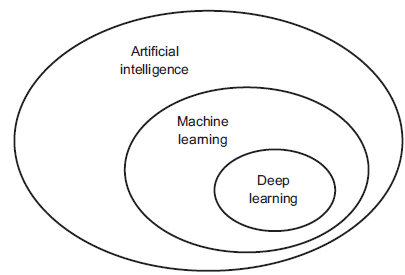Deep learning is one of many branches of machine learning, where the models are long chains of geometric functions, applied one after the other to form stacks of layers. It is one among many approaches to machine learning but not on equal footing with the others.

### What makes deep learning exceptional

Why is deep learning unequaled among machine learning techniques? Well, deep learning has achieved tremendous success in a wide range of tasks that have historically been extremely difficult for computers, especially in the areas of machine perception. This includes extracting useful information from images, videos, sound, and others.

Given sufficient training data (in particular, training data appropriately labelled by humans), it’s possible to extract from perceptual data almost anything that a human could extract. Large corporations and businesses are deriving value from deep learning by enabling human-level speech recognition, smart assistants, human-level image classification, vastly improved machine translation, and more. Google Now, Amazon Alexa, ad targeting used by Google, Baidu and Bing are all powered by deep learning. Think of superhuman Go playing and near-human-level autonomous driving.

In the summer of 2016, an experimental short movie, Sunspring, was directed using a script written by a long short-term memory (LSTM) algorithm a type of deep learning algorithm.

### How to build deep learning models

Given all this success recorded using deep learning, it's important to stress that building deep learning models is more of an art than science. To build a deep learning or any machine learning model for that matter one need to consider the following steps:

• Define the problem: What data does the organisation have? What are we trying to predict? Do we need to collect more data? How can we manually label the data? Make sure to work with domain expert because you can’t interpret what you don’t know!
• What metrics can we use to reliably measure the success of our goals.
• Prepare validation process that will be used to evaluate the model.
• Data exploration and pre-processing: This is where most time will be spent such as normalization, manipulation, joining of multiple data sources and so on.
• Develop an initial model that does better than a baseline model. This gives some indication of whether machine learning is ideal for the problem.
• Refine model architecture by tuning hyperparameters and adding regularization. Make changes based on validation data.
• Avoid overfitting.
• Once happy with the model, deploy it into production environment. This may be difficult to achieve for many organisations giving that a deep learning score code is large. This is where SAS can help. SAS has developed a scoring mechanism called "astore" which allows deep learning method to be pushed into production with just a click.

### Is the deep learning hype justified?

We're still in the middle of deep learning revolution trying to understand the limitations of this algorithm. Due to its unprecedented successes, there has been a lot of hype in the field of deep learning and AI. It’s important for managers, professionals, researchers and industrial decision makers to be able to distill this hype from reality created by the media.

Despite the progress on machine perception, we are still far from human level AI. Our models can only perform local generalization, adapting to new situations that must be similar to past data, whereas human cognition is capable of extreme generalization, quickly adapting to radically novel situations and planning for long-term future situations. To make this concrete, imagine you’ve developed a deep network controlling a human body, and you wanted it to learn to safely navigate a city without getting hit by cars, the net would have to die many thousands of times in various situations until it could infer that cars are dangerous, and develop appropriate avoidance behaviors. Dropped into a new city, the net would have to relearn most of what it knows. On the other hand, humans are able to learn safe behaviors without having to die even once—again, thanks to our power of abstract modeling of hypothetical situations.

Lastly, remember deep learning is a long chain of geometrical functions. To learn its parameters via gradient descent one key technical requirements is that it must be differentiable and continuous which is a significant constraint.

Looking beyond the AI and deep learning hype was published on SAS Users.In keras, we can visualize activation functions' geometric properties using backend functions over layers of a model.

We all know the exact function of popular activation functions such as 'sigmoid', 'tanh', 'relu', etc, and we can feed data to these functions to directly obtain their output. But how to do that via keras without explicitly specifying their functional forms?

This can be done following the four steps below:

1. define a simple MLP model with a one dimension input data, a one neuron dense network as the hidden layer, and the output layer will have a 'linear' activation function for one neuron.
2. Extract layers' output of the model (fitted or not) via iterating through model.layers
3. Using backend function K.function() to obtain calculated output for a given input data
4. Feed desired data to the above functions to obtain the output from appropriate activation function.

The code below is a demo:

``from keras.layers import Dense, Activationfrom keras.models import Sequentialimport keras.backend as Kimport numpy as npimport matplotlib.pyplot as plt``# 以下设置显示中文文方法根据 http://blog.csdn.net/rumswell/article/details/6544377 plt.rcParams['font.sans-serif'] = ['SimHei'] #指定默认字体  plt.rcParams['axes.unicode_minus'] = False #解决图像中中文符号显示为方块的问题  def NNmodel(activationFunc='linear'):    '''       定义一个神经网络模型。如果要定义不同的模型，可以直接修改该函数    '''    if (activationFunc=='softplus') | (activationFunc=='sigmoid'):        winit='lecun_uniform'    elif activationFunc=='hard_sigmoid':        winit='lecun_normal'    else:        winit='he_uniform'    model = Sequential()    model.add(Dense(1, input_shape=(1,), activation=activationFunc,                     kernel_initializer=winit,                     name='Hidden'))            model.add(Dense(1, activation='linear', name='Output'))       model.compile(loss='mse', optimizer='sgd')        return modeldef VisualActivation(activationFunc='relu', plot=True):        x = (np.arange(100)-50)/10    y = np.log(x+x.max()+1)        model = NNmodel(activationFunc = activationFunc)        inX = model.input                                              outputs = [layer.output for layer in model.layers if layer.name=='Hidden']         functions = [K.function([inX], [out]) for out in outputs]           layer_outs = [func([x.reshape(-1, 1)]) for func in functions]    activationLayer = layer_outs            activationDf = pd.DataFrame(activationLayer)    result=pd.concat([pd.DataFrame(x), activationDf], axis=1)    result.columns=['X', 'Activated']    result.set_index('X', inplace=True)    if plot:        result.plot(title=f)        return result# Now we can visualize them (assuming default settings) :actFuncs = ['linear', 'softmax', 'sigmoid', 'tanh', 'softsign', 'hard_sigmoid', 'softplus', 'selu', 'elu']from keras.layers import LeakyReLUfigure = plt.figure()for i, f in enumerate(actFuncs):    # 依次画图    figure.add_subplot(3, 3, i+1)    out=VisualActivation(activationFunc=f, plot=False)    plt.plot(out.index, out.Activated)    plt.title(u'激活函数：'+f)``
This figure is the output from above code. As we can see, the geometric property of each activation function is well captured.In many introductory to image recognition tasks, the famous MNIST data set is typically used. However, there are some issues with this data:

1. It is too easy. For example, a simple MLP model can achieve 99% accuracy, and a 2-layer CNN can achieve 99% accuracy.

2. It is over used. Literally every machine learning introductory article or image recognition task will use this data set as benchmark. But because it is so easy to get nearly perfect classification result, its usefulness is discounted and is not really useful for modern machine learning/AI tasks.

Therefore, there appears Fashion-MNIST dataset. This dataset is developed as a direct replacement for MNIST data in the sense that:

1. It is the same size and style: 28x28 grayscale image
2. Each image is associated with 1 out of 10 classes, which are:
0:T-shirt/top,
1:Trouser,
2:Pullover,
3:Dress,
4:Coat,
5:Sandal,
6:Shirt,
7:Sneaker,
8:Bag,
9:Ankle boot
3. 60000 training sample and 10000 testing sample Here is a snapshot of some samples:
Since its appearance, there have been multiple submissions to benchmark this data, and some of them are able to achieve 95%+ accuracy, most noticeably Residual network or separable CNN.
I am also trying to benchmark against this data, using keras. keras is a high level framework for building deep learning models, with selection of TensorFlow, Theano and CNTK for backend. It is easy to install and use. For my application, I used CNTK backend. You can refer to this article on its installation.

Here, I will benchmark two models. One is a MLP with layer structure of 256-512-100-10, and the other one is a VGG-like CNN. Code is available at my github: https://github.com/xieliaing/keras-practice/tree/master/fashion-mnist

The first model achieved accuracy of [0.89, 0.90] on testing data after 100 epochs, while the latter achieved accuracy of >0.94 on testing data after 45 epochs. First, read in the Fashion-MNIST data:
``import numpy as npimport io, gzip, requeststrain_image_url = "http://fashion-mnist.s3-website.eu-central-1.amazonaws.com/train-images-idx3-ubyte.gz"train_label_url = "http://fashion-mnist.s3-website.eu-central-1.amazonaws.com/train-labels-idx1-ubyte.gz"test_image_url = "http://fashion-mnist.s3-website.eu-central-1.amazonaws.com/t10k-images-idx3-ubyte.gz"test_label_url = "http://fashion-mnist.s3-website.eu-central-1.amazonaws.com/t10k-labels-idx1-ubyte.gz"def readRemoteGZipFile(url, isLabel=True):    response=requests.get(url, stream=True)    gzip_content = response.content    fObj = io.BytesIO(gzip_content)    content = gzip.GzipFile(fileobj=fObj).read()    if isLabel:        offset=8    else:        offset=16    result = np.frombuffer(content, dtype=np.uint8, offset=offset)        return(result)train_labels = readRemoteGZipFile(train_label_url, isLabel=True)train_images_raw = readRemoteGZipFile(train_image_url, isLabel=False)test_labels = readRemoteGZipFile(test_label_url, isLabel=True)test_images_raw = readRemoteGZipFile(test_image_url, isLabel=False)train_images = train_images_raw.reshape(len(train_labels), 784)test_images = test_images_raw.reshape(len(test_labels), 784)``
Let's first visual it using tSNE. tSNE is said to be the most effective dimension reduction tool.This plot function is borrowed from sklearn example.
``from sklearn import manifoldfrom time import timeimport matplotlib.pyplot as pltfrom matplotlib import offsetboxplt.rcParams['figure.figsize']=(20, 10)# Scale and visualize the embedding vectorsdef plot_embedding(X, Image, Y, title=None):    x_min, x_max = np.min(X, 0), np.max(X, 0)    X = (X - x_min) / (x_max - x_min)    plt.figure()    ax = plt.subplot(111)    for i in range(X.shape):        plt.text(X[i, 0], X[i, 1], str(Y[i]),                 color=plt.cm.Set1(Y[i] / 10.),                 fontdict={'weight': 'bold', 'size': 9})    if hasattr(offsetbox, 'AnnotationBbox'):        # only print thumbnails with matplotlib > 1.0        shown_images = np.array([[1., 1.]])  # just something big        for i in range(X.shape):            dist = np.sum((X[i] - shown_images) ** 2, 1)            if np.min(dist) < 4e-3:                # don't show points that are too close                continue            shown_images = np.r_[shown_images, [X[i]]]            imagebox = offsetbox.AnnotationBbox(                offsetbox.OffsetImage(Image[i], cmap=plt.cm.gray_r),                X[i])            ax.add_artist(imagebox)    plt.xticks([]), plt.yticks([])    if title is not None:        plt.title(title)``

tSNE is very computationally expensive, so for impatient people like me, I used 1000 samples for a quick run. If your PC is fast enough and have time, you can run tSNE against the full dataset.
``sampleSize=1000samples=np.random.choice(range(len(Y_train)), size=sampleSize)tsne = manifold.TSNE(n_components=2, init='pca', random_state=0)t0 = time()sample_images = train_images[samples]sample_targets = train_labels[samples]X_tsne = tsne.fit_transform(sample_images)t1 = time()plot_embedding(X_tsne, sample_images.reshape(sample_targets.shape, 28, 28), sample_targets,               "t-SNE embedding of the digits (time %.2fs)" %               (t1 - t0))plt.show()``
We see that several features, including mass size, split on bottom and semetricity, etc, separate the categories. Deep learning excels here because you don't have to manually engineering the features but let the algorithm extracts those.

In order to build your own networks, we first import some libraries
``from keras.models import Sequentialfrom keras.layers.convolutional import Conv2D, MaxPooling2D, AveragePooling2Dfrom keras.layers.advanced_activations import LeakyReLUfrom keras.layers import Activation``
We also do standard data preprocessing:
``X_train = train_images.reshape(train_images.shape, 28, 28, 1).astype('float32')X_test = test_images.reshape(test_images.shape, 28, 28, 1).astype('float32')X_train /= 255X_test /= 255X_train -= 0.5X_test -= 0.5X_train *= 2.X_test *= 2.Y_train = train_labelsY_test = test_labelsY_train2 = keras.utils.to_categorical(Y_train).astype('float32')Y_test2 = keras.utils.to_categorical(Y_test).astype('float32')``
Here is the simple MLP implemented in keras:
``mlp = Sequential()mlp.add(Dense(256, input_shape=(784,)))mlp.add(LeakyReLU())mlp.add(Dropout(0.4))mlp.add(Dense(512))mlp.add(LeakyReLU())mlp.add(Dropout(0.4))mlp.add(Dense(100))mlp.add(LeakyReLU())           mlp.add(Dropout(0.5))mlp.add(Dense(10, activation='softmax'))mlp.compile(loss='categorical_crossentropy', optimizer='adam', metrics=['accuracy'])mlp.summary()``

This model achieved almost 90% accuracy on test dataset at about 100 epochs. Now, let's build a VGG-like CNN model. We use an architecture that is similar to VGG but still very different. Because the figure data is small, if we use original VGG architecture, it is very likely to overfit and won't perform very well in testing data which is observed in publically submitted benchmarks listed above. To build such a model in keras is very natural and easy:
``num_classes = len(set(Y_train))model3=Sequential()model3.add(Conv2D(filters=32, kernel_size=(3, 3), padding="same",           input_shape=X_train.shape[1:], activation='relu'))model3.add(Conv2D(filters=64, kernel_size=(3, 3), padding="same", activation='relu'))model3.add(MaxPooling2D(pool_size=(2, 2)))model3.add(Dropout(0.5))model3.add(Conv2D(filters=128, kernel_size=(3, 3), padding="same", activation='relu'))model3.add(Conv2D(filters=256, kernel_size=(3, 3), padding="valid", activation='relu'))model3.add(MaxPooling2D(pool_size=(3, 3)))model3.add(Dropout(0.5))model3.add(Flatten())model3.add(Dense(256))model3.add(LeakyReLU())model3.add(Dropout(0.5))model3.add(Dense(256))model3.add(LeakyReLU())#model2.add(Dropout(0.5))model3.add(Dense(num_classes, activation='softmax'))model3.compile(loss='categorical_crossentropy', optimizer='adam', metrics=['accuracy'])model3.summary()``
This model has 1.5million parameters. We can call 'fit' method to train the model:
``model3_fit=model3.fit(X_train, Y_train2, validation_data = (X_test, Y_test2), epochs=50, verbose=1, batch_size=500)``
After 40 epochs, this model archieves accuracy of 0.94 on testing data.Obviously, there is also overfitting problem for this model. We will address this issue later.深度学习是目前最热门的高级分析技术之一，在很多方面表现出了超越传统机器学习方法的有效性。但是在常用的TensorFlowCNTKTheano等计算环境中实现不同的深度学习模型仍然需要耗费很多时间来编写程序。KERAS的出现提供了一个高度抽象的环境来搭建深度学习模型，特别是其简单易用，跟网络结构一一对应的特点使得其迅速在数据科学家这个使用人群中流行起来。

## 什么是KERAS

1. 设计初衷就是方便以模块化地方式快速构造深度学习模型的原型；

2. 可以很方便地在CPU和GPU之间切换

3. KERAS本身只是描述模型的环境，其计算平台目前依赖于TensorFlow，CNTK和Theano这三种，以后会拓展到其他流行的计算平台上，比如mxNet等；

4. KERAS的拓展性既可以通过自定义KERAS里的激活函数或者损失函数等能自定义的部分进行，也可以通过引用对应的计算平台的自定义部分进行，具有一定的灵活性；

1. 首先要将原始数据处理成KERAS的API能够接受的格式，一般是一个张量的形式，通常在维度上表示为（批量数，[单一样本对应张量的维度]）。这里[单一样本对应张量的维度] 是一个通用的说法，对应于不同类型的模型，数据有不同的要求。 通常，如果是一个简单的全链接模型，则单一样本对应张量的维度就是特征个数； 如果是一维的时间序列数据，并要用循环神经网络模型训练的话，则单一样本对应张量的维度是时间步和每个时间步对应的回看序列长度； 如果输入数据是图像，并使用卷积神经网络模型进行训练，则单一样本张量对应图像的高，宽和色彩频道三个维度。但是如果是使用全连接模型训练图像数据，则单一样本对应张量是该图像扁化（Flatten）以后的向量长度，其为高，宽和色彩频道各个维度数量的乘积。一般卷积神经网络最靠近输出层的那层都设置一个全连接层，因此也需要扁化输入张量。

2. 其次要构造需要的深度学习模型。这一步又分为模型的选择和模型的细化两个步骤：
- 选择模型的类型。KERAS里定义了两大类模型
1）序列模型(Sequential)；
2）通用模型(Model)。

序列模型指的是深度模型每一层之间都是前后序列关系，如下图所示：
Figure 1。MLP是一个典型的序列模型，[图片来源](http://article.sapub.org/10.5923.j.ajis.20120204.01.html) 可以看到从左到右，输入层到隐含层到输出层每一层之间都是前后依次相连的简单关系。这个简单的网络结构可以用三句KERAS命令实现：
``    model=Sequential()    model.add(Dense(5, input_shape=(4,), activation=’sigmoid’))    model.add(Dense(1, activation=’sigmoid’))``

``    user_in = Input(shape=(1,), dtype='int64', name='user_in')    u = Embedding(n_users, n_factors, input_length=1)(user_in)    movie_in = Input(shape=(1,), dtype='int64', name='movie_in')    v = Embedding(n_movies, n_factors, input_length=1)(movie_in)    x = merge([u, v], mode='dot')    x = Flatten()(x)    model = Model([user_in, movie_in], x)    model.compile(Adam(0.001), loss='mse')``

Figure 2。矩阵分解的深度学习模型

- 细化模型的结构。其实上面已经展示了模型结构细化之后的情况。一般来说，确定了模型类型以后，其结构不外乎每层的类型是什么，是全连接层还是卷积层还是放弃（Dropout）层；每层的其他参数是什么，比如如果需要指定激活函数，那么使用什么样的激活函数，如果是卷积层，那么需要多少过滤器，每个过滤器的大小是怎样的？等等这些都可以通过设定不同的参数进行细化。

3. 然后对模型进行编译，编译完成以后可以查看模型的基本信息，特别是参数的数量；

4. 最后带入数据对模型进行拟合。一般来讲，如果数据是静态的张量数据，通过使用fit方法。如果数据特别大，可是使用可迭代的data generator对象，并使用fit_generator方法来拟合。

## KERAS和深度学习模型的对应关系

KERAS既然是开发出来快速构造深度学习模型的工具，那么它的API和深度学习模型的要素都有很强的对应关系。 正如上面所说，目前的深度学习模型都可以纳入序列模型或者通用模型的，那么我们用图示的方式来表示这个对应关系，方便读者理解。这里网络图为了方便与按行排列的代码对应，对每一层都进行了标注。 下图展示的是一个典型的全连接序列模型：
Figure 3。全连接序列模型，修改自[博客](http://www.samyzaf.com/ML/pima/pima.html)

这个序列模型可以使用如下的KERAS命令快速搭建：
``Model = Sequential()Model.add(Dense(10, activation=’sigmoid’,                    input_shape=(8, ))             【隐含层1+输入层】Model.add(Dense(8, activation=’relu’))          【隐含层2】Model.add(Dense(10, activation=’relu’))         【隐含层3】Model.add(Dense(5, activation=’softmax’))       【输出层】``

``x = Input(shape=(8,))                          【输入层】b = Dense(10, activation=’sigmoid’)(x)       【隐含层1】c = Dense(8, activation=’relu’)(b)           【隐含层2】d = Dense(10, activation=’relu’)(c )         【隐含层3】out = Dense(5, activation=’softmax’)(d)      【输出层】model = Model(inputs=x, outputs=out)``

Figure 4。亚马逊的推荐页面局部

推荐系统的最终目的是从百万甚至上亿内容或者商品中把有用的东西高效地显示给用户，这样可以为用户节省很多自行查询的时间，也可以提示用户可能忽略的内容或商品，使用户更有黏性，更愿意花时间待在网站上，从而使商家可以从内容或者商品中赚取更多的利润，即使流量本身也会使商家从广告中受益。

传统上，推荐系统是基于矩阵分解的协同过滤算法，前面也展示了这样的一个简单模型。下面我们着重介绍深度学习推荐系统。这个模型除了能将用户和可选产品联系起来意外，还能将其他辅助数据，比如用户年龄，地区，上网设备以及各种产品属性，联系起来。

Figure 5。深度模型

``k = 128model1 = Sequential()model1.add(Embedding(n_users + 1, k, input_length = 1))model1.add(Reshape((k,)))model2 = Sequential()model2.add(Embedding(n_movies + 1, k, input_length = 1))model2.add(Reshape((k,)))``

``model = Sequential()model.add(Merge([model1, model2], mode = 'concat'))``

``model.add(Dropout(0.2))model.add(Dense(k, activation = 'relu'))model.add(Dropout(0.5))model.add(Dense(int(k/4), activation = 'relu'))model.add(Dropout(0.5))``

``model.add(Dense(int(k/16), activation = 'relu'))model.add(Dropout(0.5))``

``model.add(Dense(1, activation = 'linear'))``

``model.compile(loss = 'mse', optimizer = "adam")``

``users = ratings['user_id'].valuesmovies = ratings['movie_id'].valuesX_train = [users, movies]``

``model.fit(X_train, y_train, batch_size = 100, epochs = 50)``

##使用KERAS构造图像识别系统

Figure 6。卷积神经网络结构。来源于CNTK教程

- 输入层的图像；
- 卷积操作；
- 激活函数的应用；
- 池化操作；
- 将数据展平（Flatten），为输出到全连接层做准备；
- 全连接层准备输出；
- softmax应用于分类问题的全连接层作为输出层。

- 首先，这是一个序列模型，因此先要声明一个序列模型的对象：
model.=sequential()

- 卷积是应用一个局部的过滤器到原始数据的过程，比如下图就展示了一个3x3的过滤器应用在一个7x7的图像上过程。假设在当前步，这个过滤器的权重经过学习得到如图所示的权重结果，在当前步继续进行卷积操作就是将这个3x3的过滤器从左上角每次要么向右要么向下移动一格，和对应的图像局部的3x3的区域进行依元素点乘求和得到卷积的结果。因为依次移动，到最边上的时候过滤器会超出图像的边界，一般会将这些对应的卷积结果删除，从而卷积后的张量维度会少于原始图像。比如这个例子中原图为7x7，使用一个3x3的过滤器卷积之后最后两列和两行进行卷积的时候会使过滤器超过边界，因此最后的卷积结果是一个5x5的图像。这里可以使用多个过滤器，每个过滤器应用一次，每次应用产生的卷积结果构成隐含层的一层。比如采用16个过滤器，如果不删除边界的过滤结果，则得到新的[7x7x16]的张量。
Figure 7．卷积演示，来源于CNTK教程

1. 首先指定过滤器数量 filter，是一个整数；
2. 第二是要指定二维过滤器的大小，比如(3,3)；
3. 第三要指定步长，即延某轴移动的时候是依次移动一个像素还是多个像素，默认为1；
5. 最后，如果卷积层是第一层，那么还需要指明输入数据的维度input\_shape。因为一般用TensorFlow或者CNTK做后台，输入数据要求是channel_last，因此输入的原始维度为[样本量，高，宽，频道]，那么这里的维度只需要去掉样本量即可，即为[高，宽，频道数]，一般用X.shape[1:]即可得到。
对于上面的例子，KERAS里的典型写法是：
``model.add(Conv2D(filters=16, kernel_size=(3, 3),                  strides=1, padding=”valid”,                   input_shape=xtrain.shape[1:]))``
- 再次要添加激活层引入激活函数，通常是一个非线性的函数。激活函数既可以通过在Conv2D里面指定activation=参数引入，也可以通过单独添加Activation层引入。

如果是通过参数，则上面的代码改写为：
``model.add(Conv2D(filters=16, kernel\_size=(3, 3),                 strides=1, padding=”valid”,                  activation=’relu’)``
如果通过添加激活层引入，则在上面的代码后添加：
``model.add(Activation(‘relu’))``
- 然后进行的池化操作是在卷积神经网络中对图像特征的一种处理，通常在卷积操作和激活函数之后进行。池化操作是将原有输入按照一定大小切分成互不交叉的局部区域，目的是为了计算特征在局部的充分统计量，从而降低总体的特征数量，防止过度拟合和减少计算量。下图展示了最大池化方法的应用。在一个6x6的图像上应用3x3的池化操作，将原输入矩阵切割为不相交叉的2x2区域，每个区域的取值是对应原输入局部的最大值。
Figure 8。最大池化操作 对应于图像的最大池化层通过MaxPooling2D，KERAS也支持平均池化层，区别在于取对应局部的平均值作为池化后结果，方法为AveragePooling2D。对应上面的例子，KERAS的命令如下：
``model.add(MaxPooling2D(pool_size=(3, 3))``

- 在前面这些处理之后，但是在输出之前，通常会添加一个或者多个全连接层进一步处理数据。全连接层可以通过Dense指出，指明输出神经元个数和激活函数即可：

- 最后使用一个全连接层作为输出层，同样要求使用softmax激活函数，并使用跟输出类别同样多的神经元个数。比如识别0—9十个数字，那么就应该写作：

把所有步骤组合到一起，我们就可以将图6显示的一个卷积神经网络模型相应地写为KERAS代码了：
``model=Sequential()model.add(Conv2D(filters=32, kernel_size=(3, 3),           padding="same",           input_shape=X_train.shape[1:],           activation='relu'))model.add(MaxPooling2D(pool_size=(2, 2)))model.add(Conv2D(filters=64, kernel_size=(3, 3), padding="valid"))model.add(Activation('relu'))model.add(MaxPooling2D(pool_size=(2, 2)))model.add(Flatten())model.add(Dense(128, activation='relu'))model.add(Dense(num_classes, activation='softmax'))``

``model.compile(loss='categorical_crossentropy', optimizer='adagrad', metrics=['accuracy'])model.summary()``

Figure 9。模型信息 我们看到这个模型一共有421642个参数，大多来自于倒数第二层的全连接层。 拟合这个模型也很简单：
``model.fit(X_train, y_train,           epochs=20, verbose=1,           batch_size=10,           validation_data = (X_test, y_test))``

1. 训练用特征数据X\_train，
2. 训练用结果数据y\_train，
3. 迭代次数epochs，
4. 批量大小用batch\_size指定，
5. verbose表示显示训练过程的信息，如果值为0不显示任何中间信息，如果为1显示按批量拟合的进度，如果为2则显示拟合结果信息。
6. 另外读者还可以指定验证数据集，用validation_data这个参数表示，其包含一个tuple，第一个元素是验证用特征数据，第二个是验证用结果数据。

* 首先将数据重塑为[样本数，高，宽，色彩通道数]的格式。这个可以通过numpy.reshape方法来实现。因为keras自带的MNIST数据已经是numpy的多维矩阵，并且是单色的，因此色彩通道数为1，因此数据重塑可以用下面的命令实现。读者可自行重塑验证用数据。
``X_train = X_train.reshape(X_train.shape,                           X_train.shape,                           X_train.shape, 1).astype(float)``

* 其次，需要将数据的取值压缩到[0， 1]之间。这有利于拟合时用的随机梯度递降算法的稳定和收敛。这可以使用X_train /= 255.0 来实现。 。

* 最后要将结果数据变为One Hot Code形式。KERAS提供了一个非常方便的方法to_categorical来实现这个功能：
``y_train = keras.utils.to_categorical(y_train, len(set(y_train))) ``

Figure 10。简单卷积模型在MNIST数据上的拟合结果。

使用KERAS可以非常方便的构造自己的卷积神经网络，对于比较复杂的情况，也可以使用已经训练好的一些常见的高效模型，比如VGG16，Xception 等做迁移训练来拟合自己的数据。
Figure 11． VGG16结构，来源于：https://www.cs.toronto.edu/~frossard/post/vgg16

``model_vgg = VGG16(include_top = False,                   weights = 'imagenet',                   input_shape =(224,224,3))model = Flatten(name = 'flatten')(model_vgg.output)model = Dense(10, activation = 'softmax')(model)model_vgg_mnist = Model(model_vgg.input, model,                         name = 'vgg16')``

## 使用KERAS构造时间序列预测模型

时间序列是在商业数据或者工程数据中经常出现的一种数据形式，是以时间为次序排列，用来描述和计量一系列过程或者行为的数据的统称。比如每天商店的收入流水或者某个工厂每小时的产品产出都是时间序列数据。一般研究的时间序列数据有两种类型。最常见的是跟踪单一的计量数据随时间变化的情况，即每个时间点上收集的数据是一个一维变量，这种是最常见的，通常的时间序列默认就是这种数据，也是本章研究的对象。另外一种时间序列数据是多个对象或者多个维度的计量数据随时间变化的情况，即每个时间点上收集的数据是一个多维变量，这种一般也被称为纵向数据（Longitudinal Data），但是不属于这里介绍的对象。

Figure 12。长江月度流量时间序列 在一般的时间序列建模中，都需要检验数据的平稳性，因为传统时间序列建模都是建立在平稳数据的假设之上。这个数据具备非常强的年度周期性。使用传统的统计技术建模的时候都需要侦测周期性，并消除之，对消除周期性之后的数据运用ARIMA模型建模。
Figure 13。长江月度流量局部和移动平滑结果

``import statsmodels.api as smfrom statsmodels.tsa.stattools import acffrom scipy import signalimport peakutils as peakacf_x, acf_ci = acf(ts, alpha=0.05, nlags=36)fs=1f, Pxx_den = signal.periodogram(acf_x, fs)index = peak.indexes(Pxx_den)cycle=(1/f[index]).astype(int)fig = plt.figure()ax0 = fig.add_subplot(111)plt.vlines(f, 0, Pxx_den)plt.plot(f, Pxx_den, marker='o', linestyle='none', color='red')plt.title("Identified Cycle of %i" % (cycle))plt.xlabel('frequency [Hz]')plt.ylabel('PSD [V**2/Hz]')plt.show()print( index, f, Pxx_den)``

Figure 14。周期图谱 很明显有一个周期为 12 个月的季节性。

1. 将数据标准化为[0，1]区间。
2. 按照LSTM的要求，将输入数据组织为[样本数，时间步，特征变量数]的三位格式来组织。
3. 定义一个LSTM深度学习模型，通常为一个序列模型对象，逐层添加LSTM层或者其他层，最后通过一个全连接层输出到输出层。
4. 最后对需要的时间段进行预测。 首先对数据进行标准化，我们使用sklearn包里的MinMaxScaler函数：
``scaler = MinMaxScaler(feature_range=(0, 1))trainstd = scaler.fit_transform(train.values.astype(float).reshape(-1, 1))teststd = scaler.transform(test.values.astype(float).reshap``

Figure 15。LSTM网络结构 （修改自CNTK Tutorial）

``def create_dataset(dataset, timestep=1, look_back=1, look_ahead=1):    from statsmodels.tsa.tsatools import lagmat    import numpy as np    ds = dataset.reshape(-1, 1)        dataX = lagmat(dataset,                    maxlag=timestep*look_back,                    trim="both", original='ex')    dataY = lagmat(dataset[(timestep*look_back):],                   maxlag=look_ahead,                   trim="backward", original='ex')    dataX = dataX.reshape(dataX.shape,                           timestep, look_back)[:-(look_ahead-1)]    return np.array(dataX), np.array(dataY[:-(look_ahead-1)])``

``lookback=1lookahead=24timestep=60trainX, trainY = create_dataset(trainstd,                         timestep=timestep,                         look_back=lookback,  look_ahead=lookahead)trainX, trainY = trainX.astype('float32'), trainY.astype('float32')truthX, truthY = create_dataset(truthstd,                         timestep=timestep,                         look_back=lookback, look_ahead=lookahead)``

``batch_size=100model = Sequential()model.add(LSTM(48, batch_size=batch_size, \input\_shape=(timestep, lookback), kernel_initializer='he_uniform'))model.add(Dropout(0.15))model.add(Dense(lookahead))model.compile(loss='mean_squared_error', optimizer='adam')``

``model.fit(trainX, trainY, epochs=20, batch_size=batch_size, verbose=1)``

Figure 16。LSTM拟合过程信息

Figure 17。LSTM拟合结果

## 小结

- 深度推荐模型，运用嵌入技术可以将不同类型的信息有机结合在一起构造一个深度神经网络推荐系统；
- 图像识别模型，运用多层卷积神经网络对图像进行切割分析，得到一个精度很好的手写数字分类器。同样的技术和模型可以直接移植到其他物体识别数据上，比如CIFAR10等。我们也介绍了运用已经训练好的现成模型进行迁移学习的手段，减少拟合的参数量，在保持一定精度的情况下提高训练速度；
- 简单时间序列预测模型，运用长短记忆（LSTM）神经网络模型来有效预测具备一定周期性的时间序列模型。一个非常简单的单层LSTM模型既可以达到定制的SARIMA模型的预测精度。

Deep learning made the headlines when the UK’s AlphaGo team beat Lee Sedol, holder of 18 international titles, in the Go board game. Go is more complex than other games, such as Chess, where machines have previously crushed famous players. The number of potential moves explodes exponentially so it wasn’t [...]

Deep learning: What’s changed? was published on SAS Voices by Colin Gray